# Hausdorff implies KC

This article gives the statement and possibly, proof, of an implication relation between two topological space properties. That is, it states that every topological space satisfying the first topological space property (i.e., Hausdorff space) must also satisfy the second topological space property (i.e., KC-space)
View all topological space property implications | View all topological space property non-implications
This article gives the statement and possibly, proof, of an implication relation between two topological space properties. That is, it states that every topological space satisfying the first topological space property (i.e., compact space) must also satisfy the second topological space property (i.e., H-closed space)
View all topological space property implications | View all topological space property non-implications

## Statement

There are three equivalent formulations:

1. Any compact subset of a Hausdorff space is closed.
2. The property of being a Hausdorff space is stronger than the property of being a KC-space
3. The property of being a compact space is stronger than the property of being a H-closed space

## Proof

Suppose$X$ is a Hausdorff space and$A$ is a closed subset of$X$ that is compact in the subspace topology. We need to show that$A$ is closed in$X$. In order to do this, it suffices to show that the complement of$A$ in$X$ is an open subset. In fact, it suffices to show that for any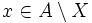$x \in A \setminus X$, there is an open subset$U$ of$X$ containing$x$, and disjoint from$A$.

Let's do this:

• For every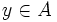$y \in A$, use the Hausdorffness of$X$ to find disjoint open subsets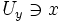$U_y \ni x$ and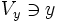$V_y \ni y$
• Now the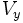$V_y$s, as$y \in A$, cover$A$, hence$V_y \cap A$ form an open cover of$A$ in the subspace topology. By compactness of$A$, we can find a finite collection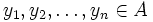$y_1, y_2, \ldots, y_n \in A$ such that the union of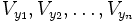$V_{y_1}, V_{y_2}, \ldots, V_{y_n}$ contains$A$
• Now consider the intersection: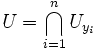$U = \bigcap_{i=1}^n U_{y_i}$

This is an open set containing$x$, because it is a finite intersection of open sets, and it is disjoint from each of the$V_{y_i}$s, hence it is disjoint from$A$. This completes the proof.

Note that the crucial thing is to pass to a finite subcover of$A$, so that we need to intersect only finitely many open subsets containing$x$, because the axioms for open subsets only guarantee that finite intersections of open subsets are open.